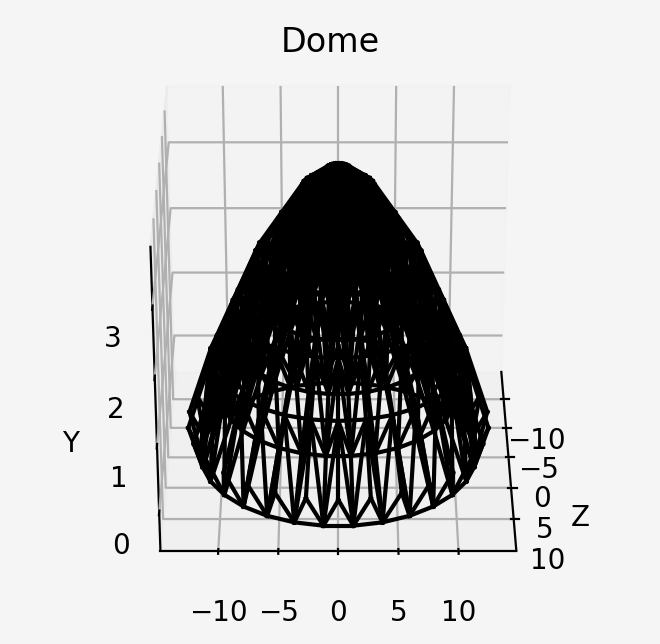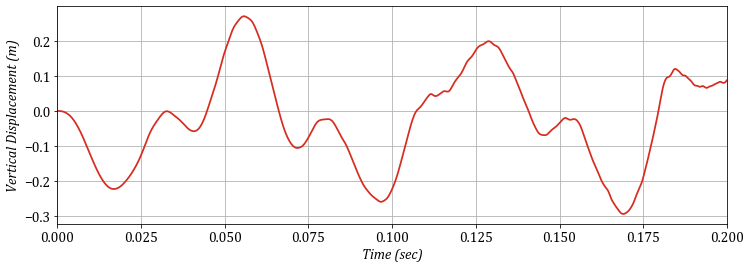# Double-Layer Shallow Dome¶

#### March 2020, Amir Hossein Namadchi¶

This is an OpenSeesPy simulation of one of the numerical examples in our previously published paper. The Core was purely written in Mathematica. This is my attempt to perform the analysis again via Opensees Core, to see if I can get the similar results. In the paper, we used Total Lagrangian framework to model the structure. Unfortunately, OpenSees does not include this framework, so, alternatively, I will use Corotational truss element.

In :
import numpy as np
import openseespy.opensees as ops
import matplotlib.pyplot as plt
import eSEESminiPy
%matplotlib notebook


Below, the base units are defined as python variables:

In :
## Units
m = 1               # Meters
KN = 1              # KiloNewtons
s = 1               # Seconds### Model Defintion¶

The coordinates information for each node are stored node_coords. Each row represent a node with the corresponding coordinates. Elements configuration are also described in connectivity, each row representing an element with its node IDs. Elements cross-sectional areas are stored in area_list. This appraoch, offers a more pythonic and flexible code when building the model. Since this is a relatively large model, some data will be read from external .txt files to keep the code cleaner.

In :
# Node Coordinates Matrix (size : nn x 3)
dtype = np.float64) * m

# Element Connectivity Matrix (size: nel x 2)
dtype = np.int64).tolist()

dtype = np.int64).tolist()

# Get Number of total Nodes
nn = len(node_coords)
# Get Number of total Elements
nel = len(connectivity)

# Cross-sectional area list (size: nel)
area_list = np.ones(nel)*(0.001)*(m**2)

# Modulus of Elasticity list (size: nel)
E_list = np.ones(nel)*(2.0*10**8)*(KN/m**2)

# Mass Density
rho = 7.850*((KN*s**2)/(m**4))

#Boundary Conditions (size: fixed_nodes x 4)
B_C = np.column_stack((np.arange(1,31),
np.ones((30,3), dtype = np.int64))
).tolist()


### Model Construction¶

I use list comprehension to add nodes,elements and other objects to the domain.

In :
ops.wipe()
ops.model('basic','-ndm',3,'-ndf',3)

# Adding nodes to the model object using list comprehensions
[ops.node(n+1,*node_coords[n]) for n in range(nn)];

# Applying BC
[ops.fix(B_C[n],*B_C[n][1:]) for n in range(len(B_C))];

# Set Material
ops.uniaxialMaterial('Elastic',1, E_list)

[ops.element('corotTruss', e+1, *connectivity[e], area_list[e],
1,'-rho', rho*area_list[e], '-cMass', 1) for e in range(nel)];


### Draw model¶

The model can now be drawn using eSEESminiPy:

In :
eSEESminiPy.drawModel()• ### Eigenvalue Analysis Let's get the first 6 periods of the structure to see if they coincide with the ones in paper.
In :
eigenvalues = ops.eigen(6)
T_list = 2*np.pi/np.sqrt(eigenvalues)
print('The first 6 period of the structure are as follows:\n', T_list)

The first 6 period of the structure are as follows:
[0.07189215 0.06809579 0.06809578 0.04648394 0.04648388 0.03117022]

• ### Dynamic Analysis Great accordance is obtained in eigenvalue analysis. Now, let's do wipeAnalysis() and perform dynamic analysis. The Newmark time integration algorithm with $\gamma=0.5$ and $\beta=0.25$ (Constant Average Acceleration Algorithm) is used. Harmonic loads are applied vertically on the loaded_nodes nodes.
In :
ops.wipeAnalysis()

P = lambda t: 250*np.sin(250*t)

# Dynamic Analysis Parameters
dt = 0.00025
time = 0.2
time_domain = np.arange(0,time,dt)

ops.timeSeries('Path', 1,
'-dt', dt,
'-values', *np.vectorize(P)(time_domain),
'-time', *time_domain)
ops.pattern('Plain', 1, 1)

# Analysis
ops.constraints('Plain')
ops.numberer('Plain')
ops.system('ProfileSPD')
ops.test('NormUnbalance', 0.0000001, 100)
ops.algorithm('ModifiedNewton')
ops.integrator('Newmark', 0.5, 0.25)
ops.analysis('Transient')

In :
time_lst =[]           # list to hold time stations for plotting
d_apex_list = []       # list to hold vertical displacments of the apex

for i in range(len(time_domain)):
is_done = ops.analyze(1, dt)
if is_done != 0:
print('Failed to Converge!')
break
time_lst.append(ops.getTime())
d_apex_list.append(ops.nodeDisp(362,3))


### Visualization¶

Below, the time history of the vertical displacement of the apex is plotted.

In :
plt.figure(figsize=(12,4))
plt.plot(time_lst, np.array(d_apex_list), color = '#d62d20', linewidth=1.75)
plt.ylabel('Vertical Displacement (m)', {'fontname':'Cambria', 'fontstyle':'italic','size':14})
plt.xlabel('Time (sec)', {'fontname':'Cambria', 'fontstyle':'italic','size':14})
plt.xlim([0.0, time])
plt.grid()
plt.yticks(fontname = 'Cambria', fontsize = 14)
plt.xticks(fontname = 'Cambria', fontsize = 14);### Closure¶

Very good agreements with the paper are obtained.

Namadchi, Amir Hossein, Farhang Fattahi, and Javad Alamatian. "Semiexplicit Unconditionally Stable Time Integration for Dynamic Analysis Based on Composite Scheme." Journal of Engineering Mechanics 143, no. 10 (2017): 04017119.